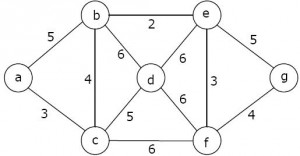Related Articles
GATE | GATE-CS-2009 | Question 38
• Last Updated : 19 Nov, 2018

Consider the following graph:Which one of the following is NOT the sequence of edges added to the minimum spanning tree using Kruskal’s algorithm?

(A) (b,e)(e,f)(a,c)(b,c)(f,g)(c,d)
(B) (b,e)(e,f)(a,c)(f,g)(b,c)(c,d)
(C) (b,e)(a,c)(e,f)(b,c)(f,g)(c,d)
(D) (b,e)(e,f)(b,c)(a,c)(f,g)(c,d)

Answer: (D)

Explanation: In the sequence (b, e) (e, f) (b, c) (a, c) (f, g) (c, d) given option D, the edge (a, c) of weight 4 comes after (b, c) of weight 3.

In Kruskal’s Minimum Spanning Tree Algorithm, we first sort all edges, then consider edges in sorted order, so a higher weight edge cannot come before a lower weight edge.

Quiz of this QuestionMy Personal Notes arrow_drop_up